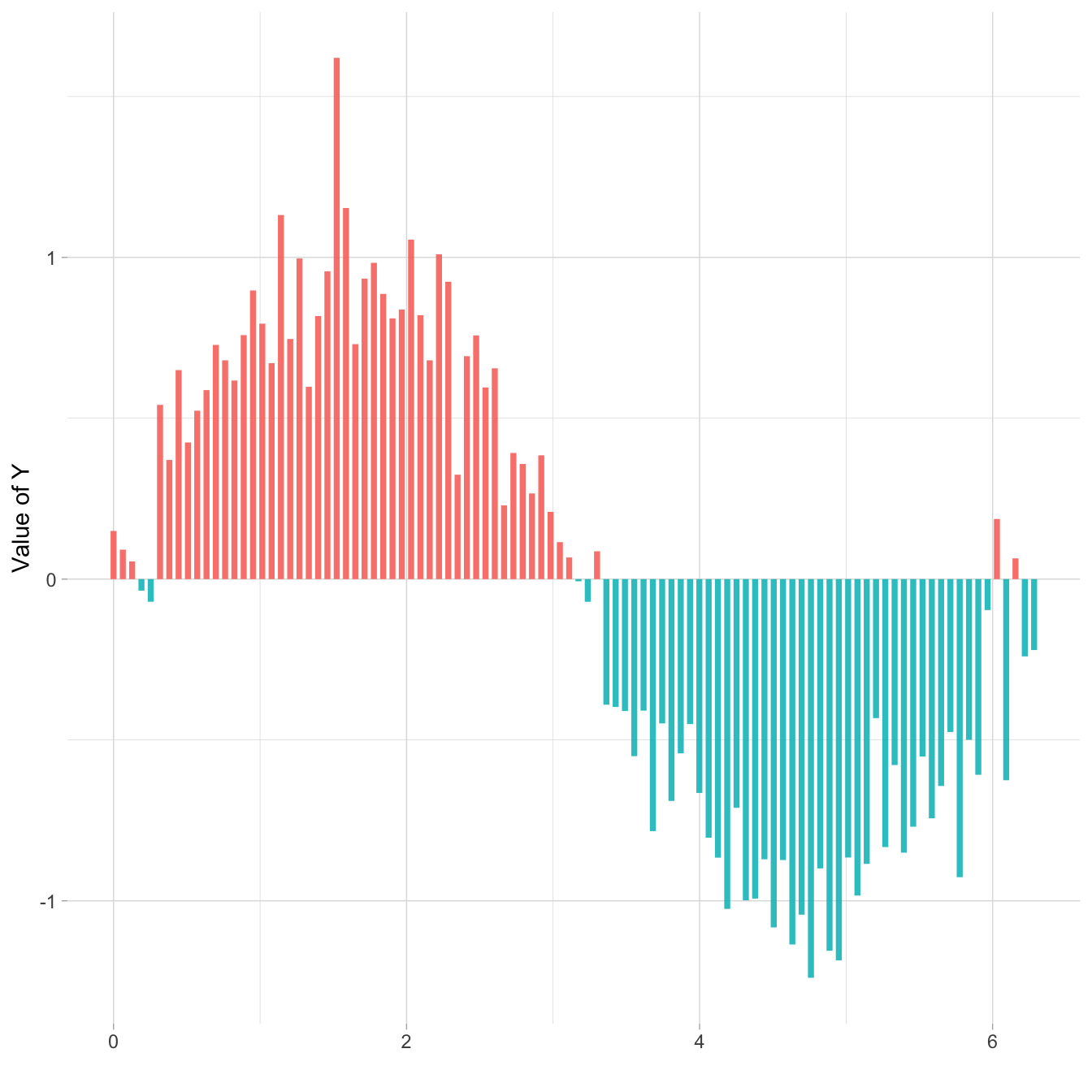# Lollipop chart with conditional color

If your lollipop plot goes on both side of an interesting threshold, you probably want to change the color of its components conditionally. Here is how using `R` and ggplot2

# Marker

Here is the process to use conditional color on your `ggplot2` chart:

• add a new column to your dataframe specifying if you are over or under the threshold (use an `ifelse` statement)
• give this column to the `color` aesthetic``````# library
library(ggplot2)
library(dplyr)

# Create data (this takes more sense with a numerical X axis)
x <- seq(0, 2*pi, length.out=100)
data <- data.frame(
x=x,
y=sin(x) + rnorm(100, sd=0.2)
)

# Add a column with your condition for the color
data <- data %>%
mutate(mycolor = ifelse(y>0, "type1", "type2"))

# plot
ggplot(data, aes(x=x, y=y)) +
geom_segment( aes(x=x, xend=x, y=0, yend=y, color=mycolor), size=1.3, alpha=0.9) +
theme_light() +
theme(
legend.position = "none",
panel.border = element_blank(),
) +
xlab("") +
ylab("Value of Y")``````

# What’s next

The lollipop chart is one of my favourite. There is so much to do with it and it is under-utilized in favor of barplot. Visit the dedicated section for more examples produced with `R`, or data-to-viz to learn about the available variations and caveats to avoid.

Related chart types

## Contact

This document is a work by Yan Holtz. Any feedback is highly encouraged. You can fill an issue on Github, drop me a message on Twitter, or send an email pasting yan.holtz.data with gmail.com.